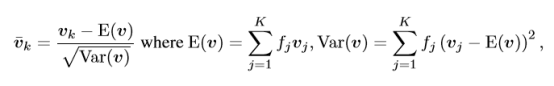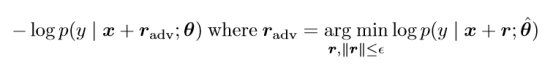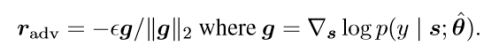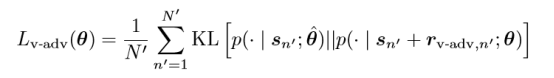Adversarial Training Methods For Semi-Supervised Text Classification

In applying the adversarial training, this paper adopts distributed word representation, or word embedding, as the input, rather than the traditional one-hot representation. The reason lies in the fact that the higher dimensionality the input has, the more likely it is to be disturbed by noise.

Introduction

This paper is the first work that applies adversarial training and virtual adversarial training to sequence models, and it greatly improved text classification tasks.

In applying the adversarial training, this paper adopts distributed word representation, or word embedding, as the input, rather than the traditional one-hot representation. The reason lies in the fact that the higher dimensionality the input has, the more likely it is to be disturbed by noise.

Adversarial training is more often used to increase the robustness of a deep learning model, by making the model less sensible to noise, or adversary. Here, since the perturbations usually don’t occur in the word embedding layer, the adversarial approach proposed by this paper is simply intended to regularize the text classifier and stabilize the classification function.

This adversarial approach proved to achieve state-of-the-art performance for multiple semi-supervised text classification tasks, including sentiment classification and topic classification.

The models are implemented on TensorFlow and the code is available at https://github.com/tensorflow/models/tree/master/adversarial_text.

Background

Experiments show that deep learning models are quite vulnerable to very small perturbations. If we add some ‘adversarial examples’ into the dataset, the deep learning models, including CNN, RNN etc., will very likely misclassify them with very high degree of confidence. In his article “Deep Learning Adversarial Examples – Clarifying Misconceptions”, Ian Goodfellow, who is also one of the co-authors of this paper, illustrated how a picture of panda could be classified as a gibbon by adding some tiny perturbation.

The reason why deep learning models are so easily influenced by adversarial examples may be the less generalization caused by overfitting and the nonlinearity of the models. However, Ian Goodfellow instead pointed out that it is just the linearity in higher-dimensional space that leads to this vulnerability.

Text Classification Model

This paper uses LSTM neural network, both unidirectional and bidirectional, as the classification model. Given a sequence of words W, its word embedding is a (K+1)*D dimentional matrix, where K is the number of words in the vocabulary. An ’end of sequence (eos)’ token is added in the word embedding as well. The LSTM model is shown as below.

In order to implement the adversarial training and virtual adversarial training, perturbations are added to the word embeddings V. But before that, one thing to note is that the perturbations are of limited norm. If the embeddings have large norm instead, the model would not be able to learn the perturbations well. As a result, some normalization steps should be done on the original word embeddings:Where f_i is the frequency of the i-th word.

The LSTM model with normalized word embeddings and perturbations is illustrated as below.

The difference between regular training and adversarial training is that the latter adds an extra term in its cost function:where r can be seen as a perturbation and epsilon is a hyperparameter limiting the size of the perturbation. Note that here, the worst situation is considered at each step, that is to say, we choose what interferes with the model the most as r at each step. Then, we train the model by minimizing the cost function above with respect to theta.

The authors further proposed an approximated calculation of r which makes it easier for us to compute the perturbation r by using backpropagation algorithm:The cost function of virtual adversarial training is distinctive, because it doesn’t require the label y. That’s why it is widely used in semi-supervised or unsupervised learning tasks. Similar to adversarial training, it is also trivial to calculate the cost function directly, but there has also been approximation approach proposed to simplify the calculation.

The cost function can be written as:where KL(p||q) refers to ‘Kullback–Leibler divergence’, also called relative entropy, which describes the difference between two probability distribution.

Back to text classification

If we denote s as a concatenation of a sequence of word embedding vectors, then the probability that we want to compute becomes p(y|s;θ) . Accordingly, adversarial perturbation r_adv on s becomes:And the adversarial loss as:where N refers to the number of labeled training examples. What we do in the experiment is to minimize the L with stochastic gradient descent.

For virtual adversarial training, we also calculated the approximated perturbation r first at each time step as:where d is a random vector.

Then the cost function becomes:where N’ refers to both labeled and unlabeled training examples.

Experiment Configuration

• Dataset

This paper tests the classifier on 5 different text datasets shown below. Note that the DBpedia dataset doesn’t have unlabeled data, so it is used for supervised learning only. Besides, in the experiment, the words that only appear in one document and ‘stop-words’ are removed, in order to improve the training efficiency.

• Pre-training

This paper uses a pre-trained recurrent language model to initialize the word matrix and the LSTM parameters. For the unidirectional LSTM model, a single hidden layer is used with 1024 hidden units. The word embedding has 256 dimentions on on IMDB and 512 on the other datasets.In the opimization process, the paper uses Adam optimizer with batch size 256. The learning rate is set to be 0.001, and at each training step, the learning rate decreases at a rate of 0.9999.

In particular, in order to increase the runing speed of the model on GPU, the paper adopts truncated backpropagation up to 400 words from each end of the sequence. The paper also adopts dropout method with dropout rate as 0.5 on the word embedding layer for the purpose of regularization.For the bidirectional LSTM model, the number of hidden units becomes 256, while other settings are the same with the unidirectional LSTM. The bidirectional LSTM is tested on IMDB, Elec and RCV.

• Text Classifier

A LSTM model with adversarial and virtual adversarial training, as described in Figure 3, was used for the classification tasks. Most of the configurations are similar to the pre-training model, such as Adam opimizer and truncated backpropagation, except that an extra hidden layer is added between the softmax layer of the target y and the final output layer.

In order to make a fair comparison among different methods, adversarial or virtual adversarial training, the paper sets a baseline, in which only pre-training and embedding dropout is used.

Results

The Figure below illustrates the general tendency of the learning curve of three methods, the baseline, Adversarial and virtual adversarial.

From the figure, we can see that the model with the adversarial and virtual adversarial training has lower loss than the baseline, and virtual adversarial even maintains this lower loss when the other two methods begin to overfit later.Figure 4. Learning curves of (a) negative log likelihood, (b) adversarial loss (deﬁned in Eq.(6)) and (c) virtual adversarial loss
• Test on the IMDB dataset

Table 2 shows the performance tested on IMDB sentiment classification task. Note that the bidirectional LSTM model has the same performance as a unidirectional LSTM with virtual adversarial training.

In order to test the performance of adversarial and virtual adversarial training on word embeddings, the paper also compare the embeddings trained using each method by finding the 10 nearest neighbors to ‘good’ and ‘bad’, calculated with the cosine distance. The results are shown below.Table 3. 10 top nearest neighbors to ‘good’ and ‘bad’ with the word embeddings trained on each method
• Test on the Elec and RCV1 datasets

Note that the bidirectional models have better performance on the RCV1 dataset. That’s because RCV1 dataset has many very long sentences, which the bidirectional model could better handle.

• Test on the Rotten Tomatoes dataset

Table 5 shows that the model with only virtual adversarial training performs worse, which may be due to the very few number of labeled sentences in the Rotten Tomatoes dataset.

• Test on the DBpedia dataset

Note that DBpedia has only labeled examples, so this task is purely supervised learning. Table 6 shows that the baseline method has already achieved state-of-the-art performance, and methods proposed in this paper further improve from the baseline method.

Conclusion

The adversarial and virtual adversarial methods proposed in this paper achieve better performance than previous models not only in text classification tasks, but also in word embedding training. This may provide valuable insights for many other research topics such as machine translation and question answering system. Besides, the proposed methods could also be used in other sequential tasks such as speech and video.

Reference

 Ian J Goodfellow, Jonathon Shlens, and Christian Szegedy. Explaining and harnessing adversarial examples. In ICLR, 2015.
Source: https://arxiv.org/pdf/1412.6572.pdf

Source: https://arxiv.org/pdf/1605.07725.pdf
Paper authors: Takeru Miyato, Andrew M Dai, Ian Goodfellow
1. Preferred Networks, Inc., ATR Cognitive Mechanisms Laboratories, Kyoto University